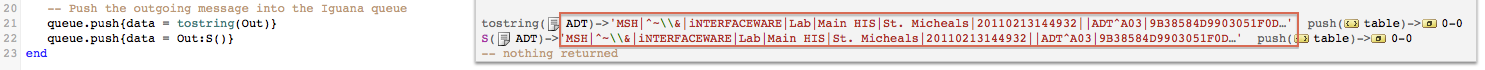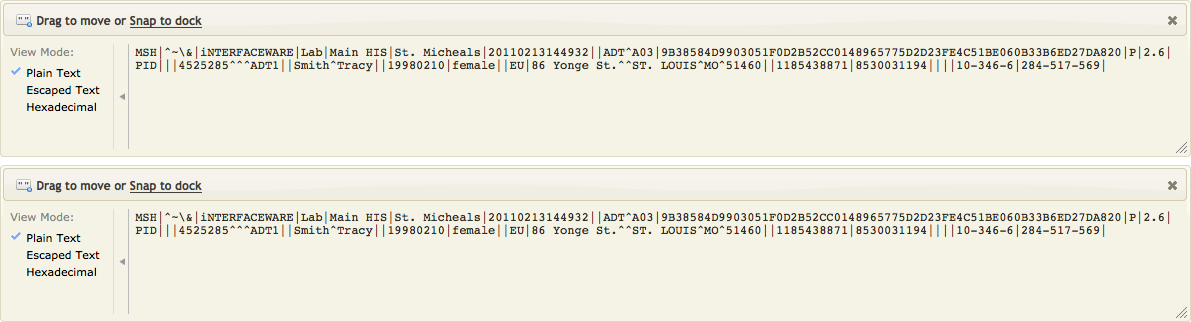# Using the S() function as shorthand for tostring()

Converting a node tree into a string is such a common task that we created the `:S()` method that you can use. The `:S()` method is simply a shorthand for the Lua `tostring()` method. All you need to do is add the node module to project, using `require()`, and you are good to go.

Note: The `:S()` function was added to the builtin node module in Iguana 5.6.16, so you only need to `require()` the node module if you are working with 5.6.15 or earlier.

Here is an example using `tostring()`: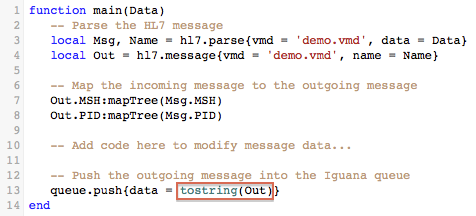Now we add the node module and use the more concise `:S()` method: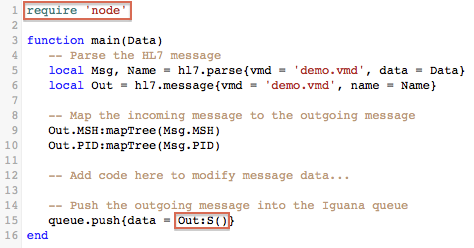If we step into the `:S()` function we can see that it simply calls `tostring()` to do the conversion: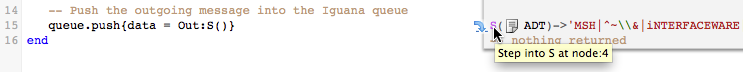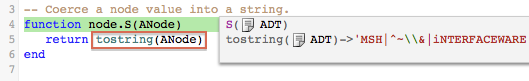If we view the annotation data from from the `queue.push{}` we can see it is the same in both cases: